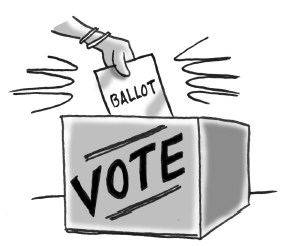### Home > INT2 > Chapter 1 > Lesson 1.3.3 > Problem1-103

1-103.Throughout this book, key problems have been selected as “checkpoints”. Each checkpoint problem is marked with an icon like the one at right. These checkpoint problems are provided so you can check to be sure you are building skills at the expected level. When you have trouble with checkpoint problems, refer to the review materials and practice problems that are available in the “Checkpoint Materials” section at the back of your book.

This problem is a checkpoint for solving problems with linear and exponential relationships. It will be referred to as Checkpoint 1.

Write and solve an equation or equations for each of the following problems.

1.In the last election, candidate C received $15,000$ fewer votes than candidate B. If a total of $109,000$ votes were cast, how many votes did candidate B receive?

2. The price of gourmet coffee beans at the corner market has increased $10 \%$ per year over the past several years. If the current cost is $14$ per pound, what did a pound of gourmet coffee beans cost two years ago?

3. Gage bought a new car for $29,000$ to use while he is away at college. The car depreciates (decreases in value) by $11\%$ annually. If he plans to sell the car once he graduates in four years, what will the value of the car be?

4. Ms. B and Ms. D are writing problems for an algebra book. Ms. B started with ten problems already written, and she can write six problems an hour. Ms. D had no problems written, but she writes ten problems an hour. When will Ms. D have the same number of problems written as Ms. B? How many problems will they each have written at that time?

Check your answers by referring to the Checkpoint 1 materials located at the back of your book.

Ideally, at this point you are comfortable working with these types of problems and can solve them correctly. If you feel that you need more confidence when solving these types of problems, then review the Checkpoint 1 materials and try the practice problems provided. From this point on, you will be expected to do problems like these correctly and with confidence.

Answers and extra practice are located in the back of your printed textbook or in the Reference Tab of your eBook. If you have an eBook for INT2, login and then click the following link: Checkpoint 1: Solving Problems with Linear and Exponential Relationships